Updating search results...

# 12 Results

View
Selected filters:
• pyramid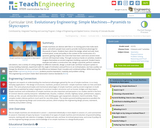Educational Use
Rating
0.0 stars

Simple machines are devices with few or no moving parts that make work easier, and which people have used to provide mechanical advantage for thousands of years. Students learn about the wedge, wheel and axle, lever, inclined plane, screw and pulley in the context of the construction of a pyramid, gaining insights into tools that have been used since ancient times and are still important today. Through numerous hands-on activities, students imagine themselves as ancient engineers building a pyramid. Student teams evaluate and select a construction site, design a pyramid, perform materials calculations, test a variety of cutting wedges on different materials, design a small-scale cart/lever transport system to convey building materials, experiment with the angle of inclination and pull force on an inclined plane, see how a pulley can change the direction of force, and learn the differences between fixed, movable and combined pulleys. While learning the steps of the engineering design process, students practice teamwork, creativity and problem solving.

Subject:
Applied Science
Engineering
Material Type:
Full Course
Provider:
TeachEngineering
Provider Set:
TeachEngineering
10/14/2015Conditional Remix & Share Permitted
CC BY-NC
Rating
0.0 stars
Rating
0.0 stars
Subject:
Mathematics
Material Type:
Full Course
Provider:
Pearson
11/02/2020Conditional Remix & Share Permitted
CC BY-NC
Rating
0.0 stars

Surface Area and Volume

Type of Unit: Conceptual

Prior Knowledge

Students should be able to:

Identify rectangles, parallelograms, trapezoids, and triangles and their bases and heights.
Identify cubes, rectangular prisms, and pyramids and their faces, edges, and vertices.
Understand that area of a 2-D figure is a measure of the figure's surface and that it is measured in square units.
Understand volume of a 3-D figure is a measure of the space the figure occupies and is measured in cubic units.

Lesson Flow

The unit begins with an exploratory lesson about the volumes of containers. Then in Lessons 2–5, students investigate areas of 2-D figures. To find the area of a parallelogram, students consider how it can be rearranged to form a rectangle. To find the area of a trapezoid, students think about how two copies of the trapezoid can be put together to form a parallelogram. To find the area of a triangle, students consider how two copies of the triangle can be put together to form a parallelogram. By sketching and analyzing several parallelograms, trapezoids, and triangles, students develop area formulas for these figures. Students then find areas of composite figures by decomposing them into familiar figures. In the last lesson on area, students estimate the area of an irregular figure by overlaying it with a grid. In Lesson 6, the focus shifts to 3-D figures. Students build rectangular prisms from unit cubes and develop a formula for finding the volume of any rectangular prism. In Lesson 7, students analyze and create nets for prisms. In Lesson 8, students compare a cube to a square pyramid with the same base and height as the cube. They consider the number of faces, edges, and vertices, as well as the surface area and volume. In Lesson 9, students use their knowledge of volume, area, and linear measurements to solve a packing problem.

Subject:
Geometry
Mathematics
Provider:
Pearson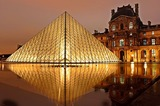Conditional Remix & Share Permitted
CC BY-NC
Rating
0.0 stars

Lesson OverviewStudents use scissors to transform a net for a unit cube into a net for a square pyramid. They then investigate how changing a figure from a cube to a square pyramid affects the number of faces, edges, and vertices and how it changes the surface area and volume.Key ConceptsA square pyramid is a 3-D figure with a square base and four triangular faces.In this lesson, the net for a cube is transformed into a net for a square pyramid. This requires cutting off one square completely and changing four others into isosceles triangles.It is easy to see that the surface area of the pyramid is less than the surface area of the cube, because part of the cube's surface is cut off to create the pyramid. Specifically, the surface area of the pyramid is 3 square units, and the surface area of the cube is 6 square units. Students will be able to see visually that the volume of the pyramid is less than that of the cube.Students consider the number of faces, vertices, and edges of the two figures. A face is a flat side of a figure. An edge is a segment where 2 faces meet. A vertex is the point where three or more faces meet. A cube has 6 faces, 8 vertices, and 12 edges. A square pyramid has 5 faces, 5 vertices, and 8 edges.Goals and Learning ObjectivesChange the net of a cube into the net of a pyramid.Find the surface area of the pyramid.

Subject:
Geometry
Material Type:
Lesson Plan
Author:
Pearson
11/02/2020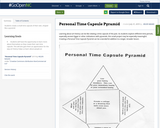Conditional Remix & Share Permitted
CC BY-NC
Rating
0.0 stars

Students create a small time capsule of their own, shaped like a pyramid.

Subject:
Arts Education
Visual Arts
Material Type:
Activity/Lab
11/06/2019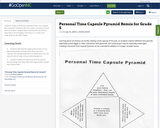Conditional Remix & Share Permitted
CC BY-NC-SA
Rating
0.0 stars

Students create a small time capsule of their own, shaped like a pyramid.
Students will also create a narrative around their art project. Imagine what everyday life would have been like in ancient Egypt.
This lesson is a remix of an original lesson by Helen Nagan

Subject:
Arts Education
Visual Arts
Material Type:
Activity/Lab
07/10/2020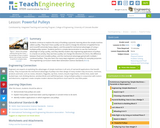Educational Use
Rating
0.0 stars

Students continue to explore the story of building a pyramid, learning about the simple machine called a pulley. They learn how a pulley can be used to change the direction of applied forces and move/lift extremely heavy objects, and the powerful mechanical advantages of using a multiple-pulley system. Students perform a simple demonstration to see the mechanical advantage of using a pulley, and they identify modern day engineering applications of pulleys. In a hands-on activity, they see how a pulley can change the direction of a force, the difference between fixed and movable pulleys, and the mechanical advantage gained with multiple / combined pulleys. They also learn the many ways engineers use pulleys for everyday purposes.

Subject:
Applied Science
Engineering
Material Type:
Activity/Lab
Lesson Plan
Provider:
TeachEngineering
Provider Set:
TeachEngineering
Author:
Denise Carlson
Jacquelyn Sullivan
Justin Fritts
Lawrence E. Carlson
Malinda Schaefer Zarske
09/18/2014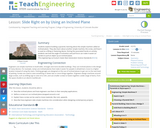Educational Use
Rating
0.0 stars

Students explore building a pyramid, learning about the simple machine called an inclined plane. They also learn about another simple machine, the screw, and how it is used as a lifting or fastening device. During a hands-on activity, students see how the angle of inclination and pull force can make it easier (or harder) to pull an object up an inclined plane.

Subject:
Applied Science
Engineering
Material Type:
Activity/Lab
Lesson Plan
Provider:
TeachEngineering
Provider Set:
TeachEngineering
Author:
Denise W. Carlson
Jacquelyn F. Sullivan
Lawrence E. Carlson
Malinda Schaefer Zarske
Travis Reilly
09/18/2014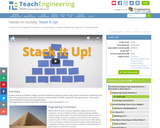Educational Use
Rating
0.0 stars

Students analyze and begin to design a pyramid. Working in engineering teams, they perform calculations to determine the area of the pyramid base, stone block volumes, and the number of blocks required for their pyramid base. They make a scaled drawing of the pyramid using graph paper.

Subject:
Applied Science
Engineering
Material Type:
Activity/Lab
Lesson Plan
Provider:
TeachEngineering
Provider Set:
TeachEngineering
Author:
Denise Carlson
Glen Sirakavit
Gregory Ramsey
Jacquelyn Sullivan
Lawrence E. Carlson
Malinda Schaefer Zarske
09/26/2008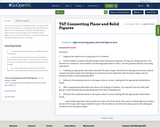Conditional Remix & Share Permitted
CC BY-NC-SA
Rating
0.0 stars

This resource is part of Tools4NCTeachers.

The goal of this lesson is for students to understand that plane figures are different from solid figures. Students will identify and locate the face of a solid figure that matches a plane figure, such as the face of a cube is a square, the base of a triangular based pyramid is a triangle.

Subject:
Mathematics
Material Type:
Lesson
Lesson Plan
11/06/2019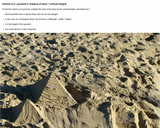Unrestricted Use
CC BY
Rating
0.0 stars

Formula for volume of pyramid. Photo of pyramid built on beach with a hotspot showing of a pyramid on geometric plane with length, base and height labeled.

Subject:
Geometry
Mathematics
Material Type:
Interactive
Provider:
Michigan Virtual
07/19/2019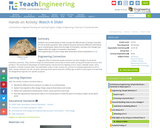Educational Use
Rating
0.0 stars

Students use inclined planes as they recreate the difficult task of raising a monolith of rock to build a pyramid. They compare the push and pull of different-sized blocks up an inclined plane, determine the angle of inclination, and learn the changes that happen when the angle is increased or decreased.

Subject:
Applied Science
Engineering
Material Type:
Activity/Lab
Provider:
TeachEngineering
Provider Set:
TeachEngineering
Author:
Denise W. Carlson
Glen Sirakavit
Jacquelyn F. Sullivan
Lawrence E. Carlson
Malinda Schafer Zarske
Travis Reilly